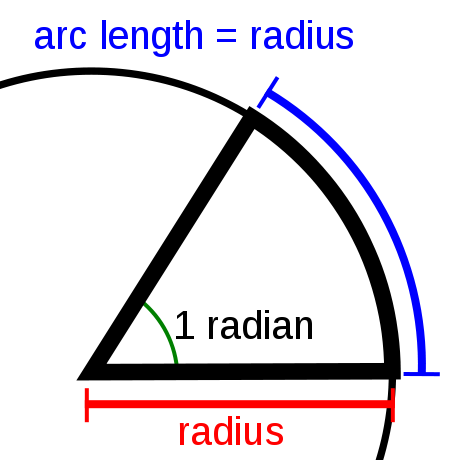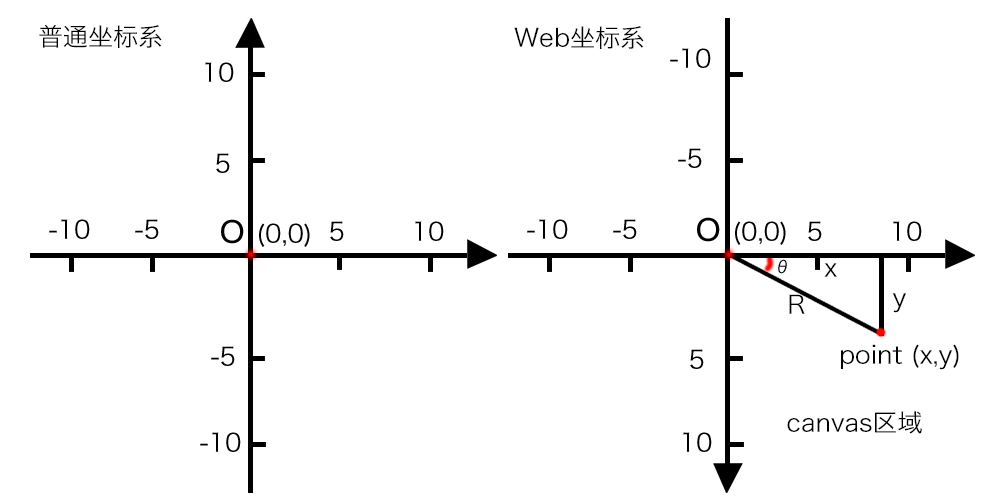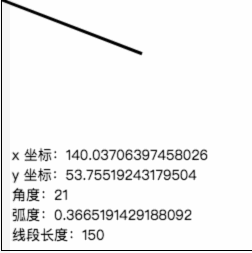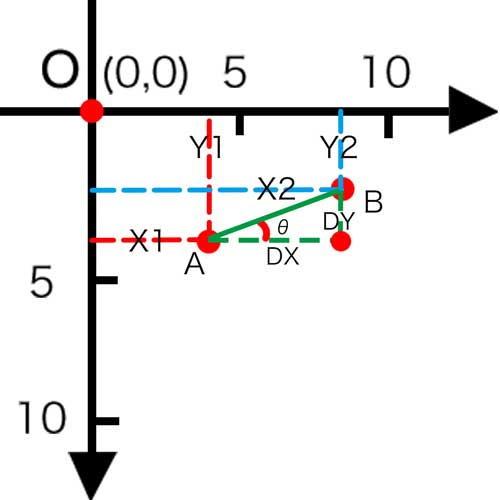# HTML5 Canvas ：三角函数的应用

## 什么是三角函数？

``````// 返回一个数值的正弦值。
Math.sin(x)

// 返回一个数值的余弦值。
Math.cos(x)

// 返回一个数值的正切值。
Math.tan(x)

// 其中参数 x 是一个表示弧度的数值
// 还有一些常用的反三角函数

// 返回一个数值的反正弦值（以弧度为单位）。
Math.asin(x)

// 返回一个数值的反余弦值（以弧度为单位）。
Math.acos(x)

// 返回一个数值的反正切值（以弧度为单位）。
Math.atan(x)

// 返回从正 x 轴到点 (x,y) 与原点连线之间的偏移角度值，这是一个逆时针角度，以弧度为单位。
Math.atan2(y, x)
``````

## 弧度与角度

``````// π，即圆的周长和它的直径之比。这个值近似为 3.141592653589793。
Math.PI
``````

“弧长等于半径的弧，其所对的圆心角为 1 弧度。”``````弧度值 = 角度值 * Math.PI / 180

``````

``````<html>
<body>
<canvas id="myCanvas" width="250" height="250" style="border: 1px solid">
你的浏览器不支持canvas,请升级你的浏览器
</canvas>
</body>
</html>
<script>
// 准备画布
var canvas = document.getElementById("myCanvas");
var ctx = canvas.getContext("2d");
var x = 40,y = 100;

ctx.lineWidth = 3;
ctx.moveTo(0,0);
ctx.lineTo(x,y);
ctx.stroke();

ctx.font = "14px sans-serif";
// 计算出结束点与原点连线与 x 轴间的弧度
// 换算出结束点与原点连线与 x 轴间的角度
var degrees = Math.atan2(y,x) * 180 / Math.PI;
// 依据定义：邻边 / 斜边 = cos (角度)
// 所以，线段的长度 = 斜边 = 邻边 / cos (角度)
var lineLength = x / Math.cos(radians)

ctx.fillText("y 坐标：" + y, 10, 180);
ctx.fillText("x 坐标：" + x, 110, 180);
ctx.fillText("角度：" + degrees, 10, 200);
ctx.fillText("线段长度：" + lineLength, 10, 240);
</script>
```````Math.atan2(y,x)` 写成 `Math.atan2(x,y)` 也可以计算出数值，但要分辨出控制点与原点的连线是与那一条轴形成的夹角才是有效夹角。

## 角度与坐标``````// 三角函数

// 反三角函数

// 参数：x 是邻边，y 是对边，R 是斜边，θ 是角度
``````

``````<html>
<body>
<canvas id="myCanvas" width="250" height="250" style="border: 1px solid">
你的浏览器不支持canvas,请升级你的浏览器
</canvas>
</body>
</html>
<script>
// 准备画布
var canvas = document.getElementById("myCanvas");
var ctx = canvas.getContext("2d");

var r = 150
var degrees = 0;

function draw (){
var radians = degrees * Math.PI / 180;

// 通过斜边计算出控制点坐标
var x = r * Math.cos(radians);
var y = r * Math.sin(radians);

// 画出原点至控制点的线
ctx.lineWidth = 3;
ctx.beginPath();
ctx.moveTo(0,0);
ctx.lineTo(x,y);
ctx.stroke();

ctx.font = "14px sans-serif";
ctx.fillText("x 坐标：" + x, 10, 160);
ctx.fillText("y 坐标：" + y, 10, 180);
ctx.fillText("角度：" + degrees, 10, 200);
ctx.fillText("线段长度：" + r, 10, 240);
}

setInterval(function(){
ctx.clearRect(0,0,250,250);
draw ();
if (degrees <=90){
degrees++;
}
},1000)
</script>
``````## 两点间的距离

``````// 两点间的 x 轴的距离
var distanceX = x2 - x1;
// 两点间的 y 轴的距离
var distanceY = y2 - y1;
// 根据勾股定理计算出斜边，也就是两点间的距离
var distance = Math.sqrt(distanceX * distanceX + distanceY * distanceY);
``````

## 两点间连线的倾斜角``````var angle = Math.atan(distanceY/distanceX);
``````

# You May Also Like